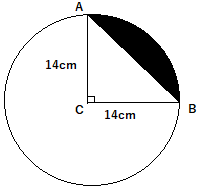mycollegehive
A chord AB of a circle of radius 14cm makes a right angle at the centre of the circle. Find the area of the minor segment.
CBSE class-10 math areas-related-to-circles0A chord AB of a circle of radius 14cm makes a right angle at the centre of the circle. Find the area of the minor segment.

18 viewsShareFollowUniversity of Lagos Nigeria
01 August 2020University of Benin Nigeria
01 August 20200Area of the segment (shaded portion) = Area of sector ACB - Area of triangle ACB

∠ACB = 90 [because it's a right angle].

Area of sector ACB = 1/2 (length of arc x radius)

length of arc = θ/360 x 2πr

Area of sector ACB = 1/2 (θ/360 x 2πr x r)

= 1/2 (θ/360 x 2πr2)

= θ/360 x πr2

r = 14cm

θ= 90

Thus, area of sector ACB = 90/360 x π x (14)2

= 49π

but, π = 22/7

Area of sector ACB = 49 x 22/7

= 154

Area of triangle ACB = 1/2 base x height

= 1/2 x 14 x 14

= 98

Therefore, area of the segment = 154 - 98

= 56 cm2Share### Related Tags

CBSE

0 followers

56 questionsclass-10

0 followers

56 questionsmath

2 followers

985 questions